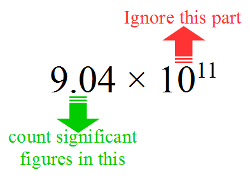Home » Significant Figures Made Easy – Step 5 of 5

# Significant Figures Made Easy – Step 5 of 5

## Miscellaneous

Some miscellaneous points and pointers relating to significant figures have been gathered in this page.

• In O Level Physics papers, answers are not accepted as fractions because examiners want to encourage students to give answers to an appropriate number of significant figures.
• If you need to determine the number of significant digits in a number that is written in scientific notation, then only consider the coefficient and ignore the base and the exponent. See below:• In case of large integers with zeroes at the end, the trailing zeroes may or may not be significant. So 543100 may be having 4 or 5 or 6 significant figures. Using scientific notation removes this ambiguity. For example, the same number 543100 shall be written in scientific notation
• with six significant digits as 5.43100 x 105
• with five significant digits as 5.4310 x 105
• with four significant digits as 5.431 x 105

…back